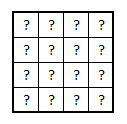# Saturday problem of combinatoricsFind the number of arrangements in a grid of $$4\times4$$ for the numbers $$1$$ to $$16$$ so that each colum and row has an odd sum.

The answer can be written as $$2^a\times3^b\times 8!^c$$. Submit your answer as $$2^a\times3^b+ 8!$$

×

Problem Loading...

Note Loading...

Set Loading...